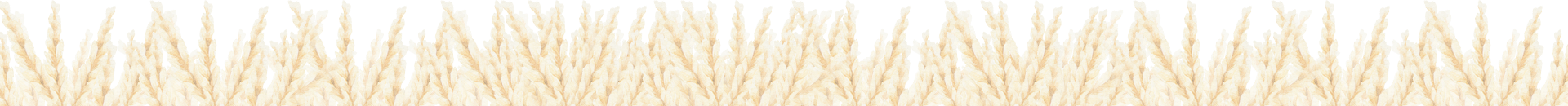### 講節氣、猜氣象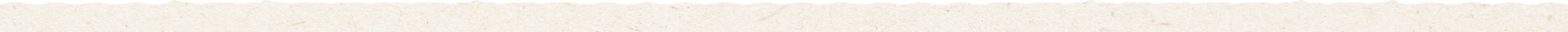#### 古聖賢不但發明二十四節氣，每個節氣都有幾句農諺，猜測氣象，以供農耕參照。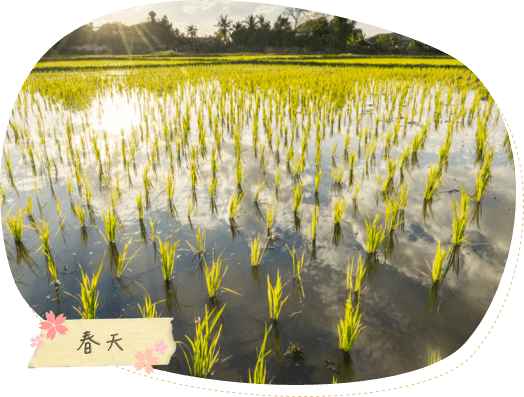• 一年之計在於春
• 春天童子面
• 春寒雨如泉
• 春到萬物蘇生
• 立春落雨透清明
• 春看海口
• 春風轉北三城去一角

• 天無落雨，農夫走無路
• 雙春雙水好年冬
• 天無落雨，大家無頭路
• 雨打上元燈，風吹清明前
• 好上元，好早冬
• 正月蔥，二月韮

• 驚蟄蚊蟲打無直
• 雷把驚蟄前，百日不開天
• 驚蟄春雷響
• 雷把驚蟄鳥仔晒羽
• 二月寒死牛，三月寒死播田夫

• 春分日夜對分
• 春分前好蒔(ㄕˊ)田，春分後好種豆
• 二、八月三種衫
• 春天冬風雨連連
• 春夏風不勝帆，秋冬帆不勝風
• 風是雨頭，屁是糞頭

• 清明時節雨紛紛
• 清明穀雨寒死虎母
• 清明掃墓節
• 清明前插竹平
• 三月天一日剝皮三日蓋被
• 春已卯風，稻尾空

• 穀雨補老母
• 穀雨天草葉青
• 穀雨天早青春
• 穀雨桂竹筍
• 穀雨桐花開
• 四月雪滿山白
• 三月杏，四月雍
• 三月肖媽祖• 立夏到夏蟬叫
• 四月一、下雨結無子
• 立夏補老爸
• 立夏梅雨到
• 夏天東風水斷源
• 立夏百病來
• 立夏小滿雨水相趕

• 四月芒種雨
• 五月無乾土
• 六月火燒埔
• 七月那烘爐
• 八月風颱雨
• 頂看初三，下看十八

• 未吃五月粽，破裘不甘放
• 飲一嘴午時，勝過吃三年補藥
• 午時水，人吃會美
• 西北雨落不過田岸
• 瓜路怕大水
• 做田錢萬萬年

• 六月雨卡冷霜
• 五月匏(ㄆㄠˊ)，六月瓜
• 夏至日頭長
• 六月起風颱
• 早日不成天
• 早看東南，晚看西北
• 夏至愛吃不愛去

• 夏至小暑，雨季中止
• 小暑小禾黃
• 空早雷，未過五時雨
• 小暑田頭黃
• 過小暑，日熱三分
• 小暑大暑無君子
• 小暑大暑無米可煮
• 小暑不見穗，大暑不見稿

• 大暑滿田光
• 小暑小熱，大暑大熱
• 早冬靠肥份，晚冬播時辰
• 一雷壓九颱
• 大暑雙冬趕，累死農夫
• 大暑無熱夠，大水風颱到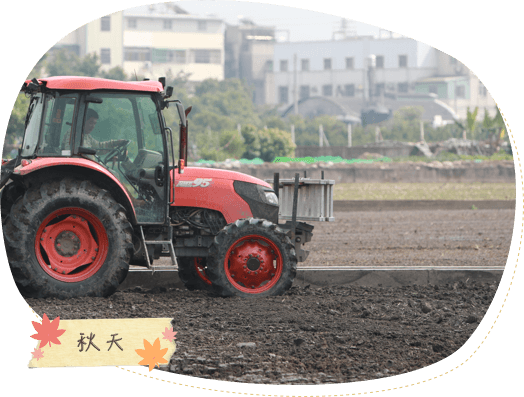• 立秋爸爸節
• 立秋趕溜溜
• 立秋半收
• 不怕七月鬼，只怕上月水
• 七月筍，八月芋
• 秋霖夜雨當過施肥
• 秋老虎 l 八月風颱雨

• 秋天東風禾白穗
• 處暑播田不夠飼老鼠
• 處暑龍眼熟

• 好中秋，好晚稻
• 白露水，毒死鬼
• 八月半，禾打盼(客語，化妝之意)
• 年怕中秋，月半半，星期最怕禮拜三
• 中秋文旦柚
• 白露雍毒過飯
• 白露水毒如鬼
• 八月半田頭看
• 吃米粉芋找好頭路
• 白露苟，一支發，一支允
• 白露雨寒露風大過三界公
• 白露不挖土

• 秋分日夜對分
• 好中秋水月娘
• 九月颱，無人知
• 四九五月無菜配

• 九月雨，貴如油
• 九月狗納日，十月日生羽
• 秋高氣爽，出穗適期
• 有風有露水，稻仔好出穗
• 十月小陽春

• 霜降，風颱走去藏
• 九月九降風
• 十月日生羽，含漫查某領不近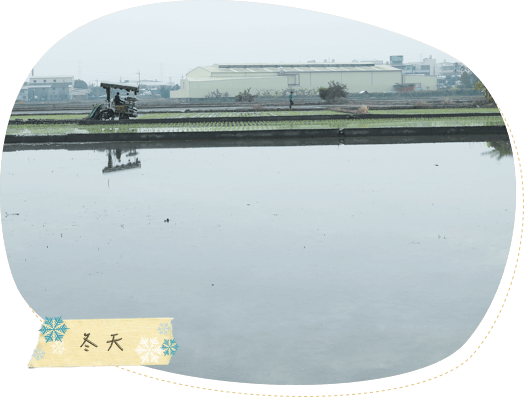• 立冬補冬，刣(ㄓㄨㄥ)鴨公補嘴空
• 立冬好收冬
• 十月小陽春
• 立冬田頭空

• 暑過秋來
• 秋收冬藏
• 早冬雨，曉冬霜

• 大雪是好年
• 大雪成堆，天下白
• 早雨早晴，晴雨留暝
• 黑寒雪凍，蟲蟄走去藏

• 冬至夜最長
• 冬至雙頭鳥
• 講冬至11月無人信
• 冬至烏過年蘇
• 冬至在月頭，寒冷在年兜
• 冬至月中央，無雪加無雙
• 冬至在月底，寒冷正二月
• 冬看山頭，春看海口
• 吃了冬至圓加一歲
• 冬至圓吃團圓

• 小寒小冷
• 十二月南風現報
• 冬寒做大旱
• 冬天東風雪滿天

• 大寒大冷
• 大寒不寒，人馬不安
• 冬寒雨四散
• 春寒雨那泉
• 冬天過了是春天